# Exploring Real Numbers About Real Numbers Real Numbers

• Slides: 26
Download presentationExploring Real Numbers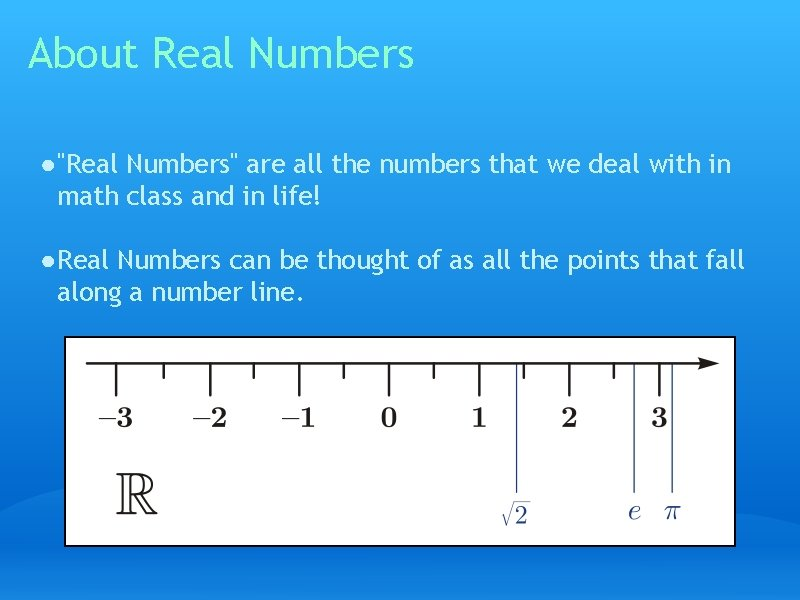About Real Numbers ● "Real Numbers" are all the numbers that we deal with in math class and in life! ● Real Numbers can be thought of as all the points that fall along a number line.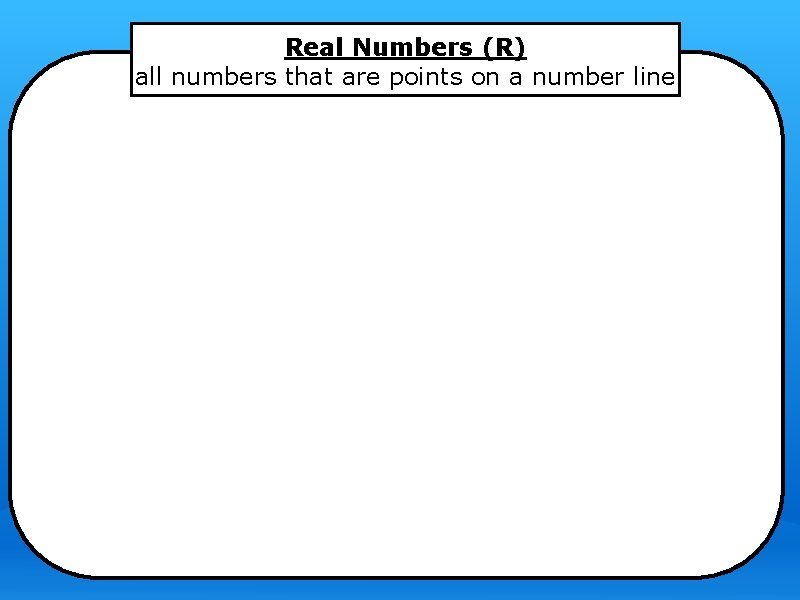Real Numbers (R) all numbers that are points on a number line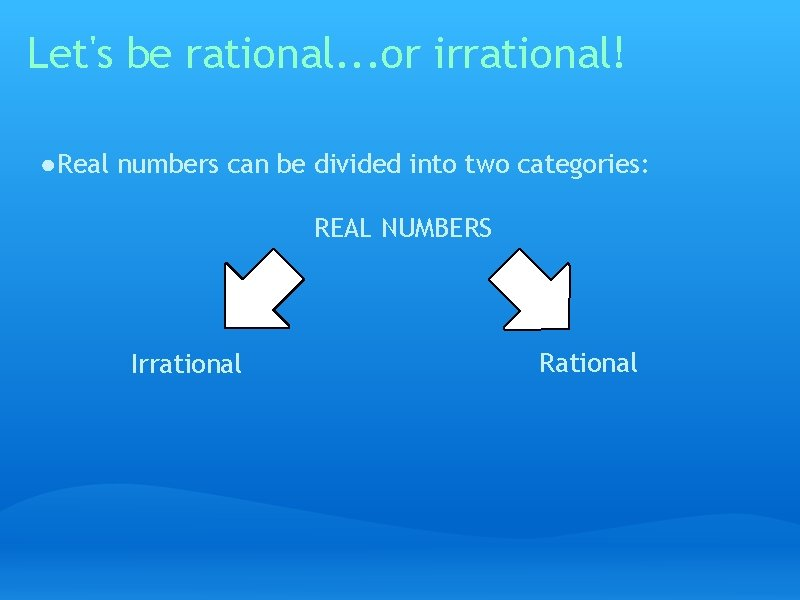Let's be rational. . . or irrational! ● Real numbers can be divided into two categories: REAL NUMBERS Irrational RationalRational Numbers (ℚ) ● any number that can be written as a fraction 1/2 3. 5 9/3 -1/3 ● any decimal that terminates (ends) or repeats 0. 325 -0. 3333333. . .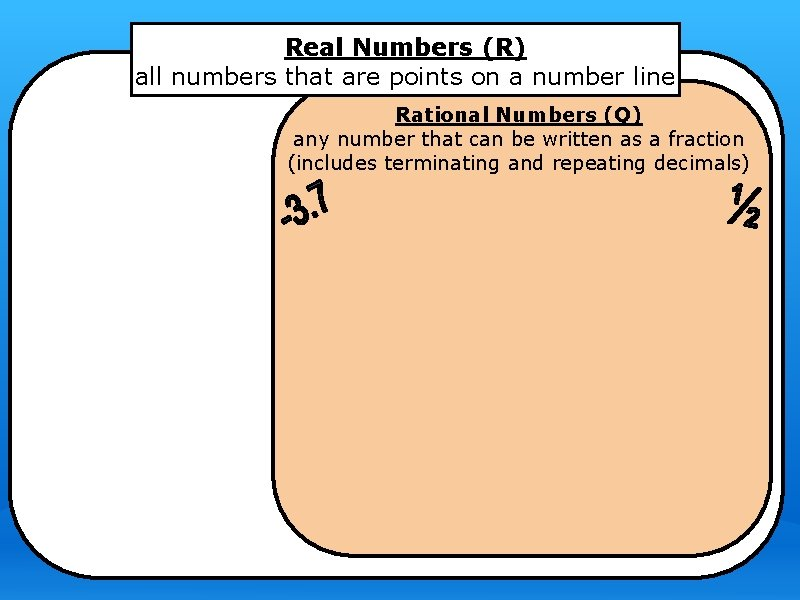Real Numbers (R) all numbers that are points on a number line Rational Numbers (Q) any number that can be written as a fraction (includes terminating and repeating decimals)Irrational Numbers (I)* ● any number that can not be written as a fraction (as a ratio of 2 whole numbers) ● a non-repeating, non-terminating decimalReal Numbers (R) all numbers that are points on a number line Irrational Numbers (I*) any number that cannot be written as a fraction. Nonterminating, nonrepeating decimals Rational Numbers (Q) any number that can be written as a fraction (includes terminating and repeating decimals)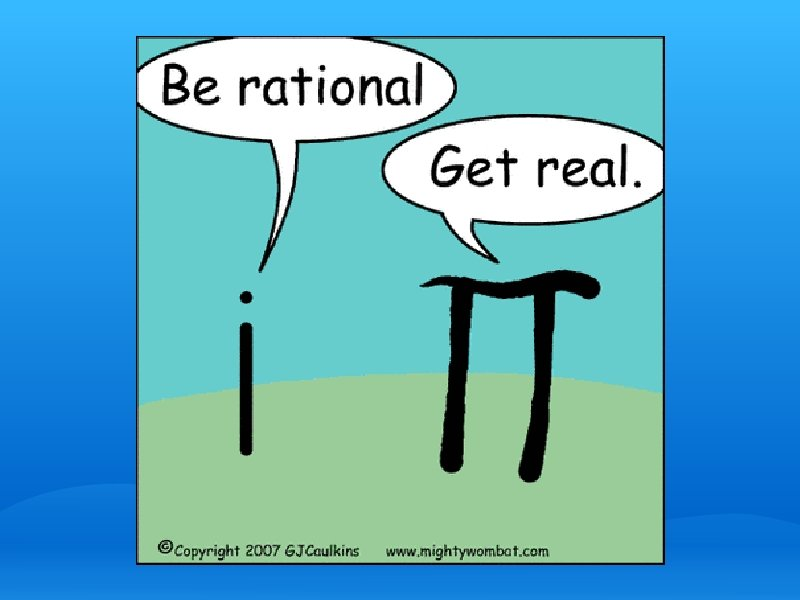Rational Number Breakdown ● Rational numbers can be divided into 3 categories REAL NUMBERS Irrational Rational Integers Whole Numbers Natural NumbersNatural Numbers (ℕ) ● Natural numbers are the counting numbers. They are probably the first numbers you learned about. 1, 2, 3, 4, 5. . .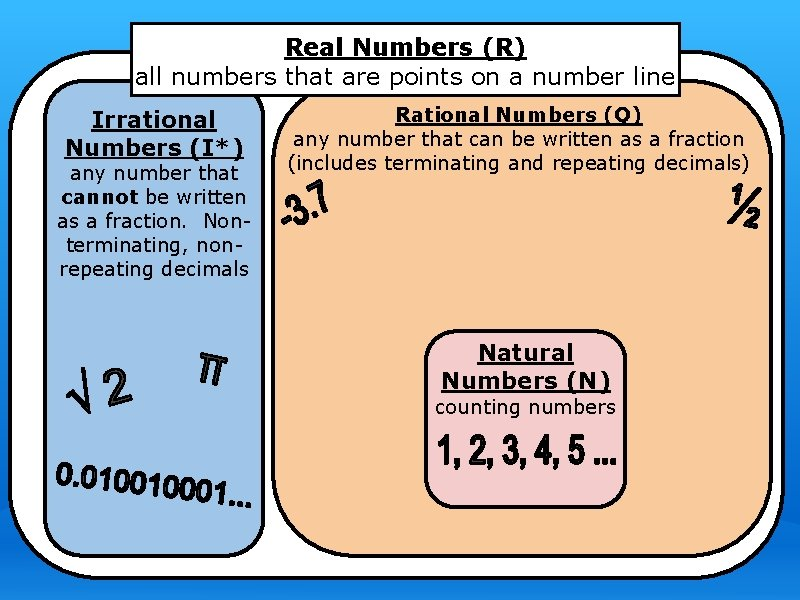Real Numbers (R) all numbers that are points on a number line Irrational Numbers (I*) any number that cannot be written as a fraction. Nonterminating, nonrepeating decimals Rational Numbers (Q) any number that can be written as a fraction (includes terminating and repeating decimals) Natural Numbers (N) counting numbersWhole Numbers (W) ● Add a zero to the Natural Numbers and you'll get the set called "Whole Numbers" 0, 1, 2, 3, 4, 5. . .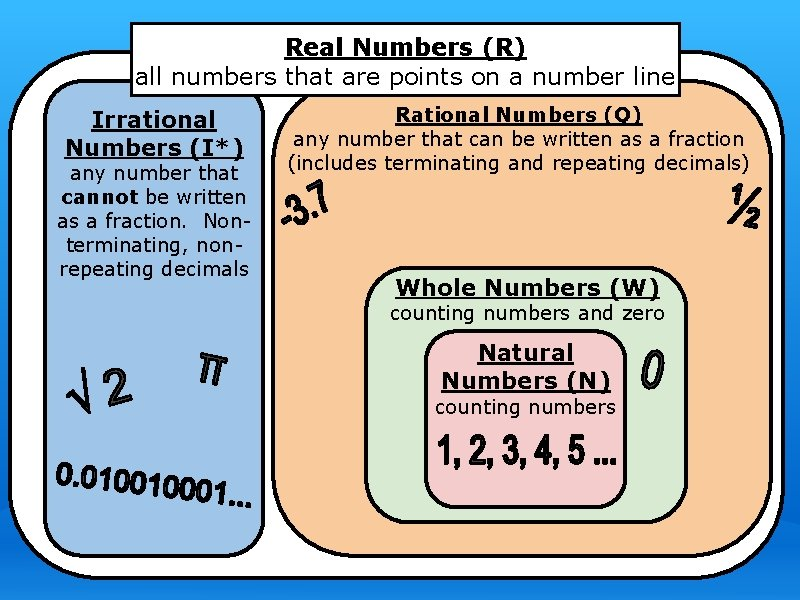Real Numbers (R) all numbers that are points on a number line Irrational Numbers (I*) any number that cannot be written as a fraction. Nonterminating, nonrepeating decimals Rational Numbers (Q) any number that can be written as a fraction (includes terminating and repeating decimals) Whole Numbers (W) counting numbers and zero Natural Numbers (N) counting numbersIntegers (ℤ) ● Integers include all counting numbers, their opposites, and zero ● The opposites of the counting numbers are simply the negatives . . . -5, -4, -3, -2, -1, 0, 1, 2, 3, 4, 5. . .Real Numbers (R) all numbers that are points on a number line Irrational Numbers (I*) any number that cannot be written as a fraction. Nonterminating, nonrepeating decimals Rational Numbers (Q) any number that can be written as a fraction (includes terminating and repeating decimals) Integers (Z) whole numbers and their opposites Whole Numbers (W) counting numbers and zero Natural Numbers (N) counting numbers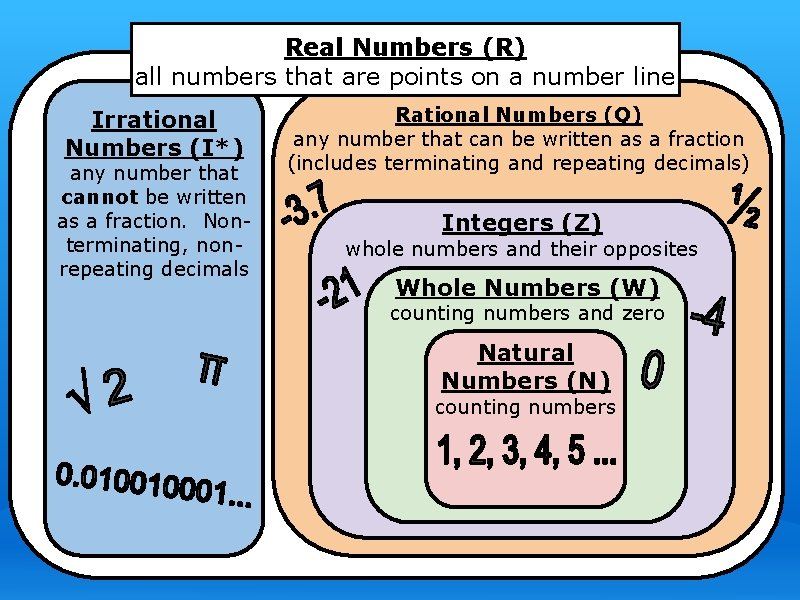Real Numbers (R) all numbers that are points on a number line Irrational Numbers (I*) any number that cannot be written as a fraction. Nonterminating, nonrepeating decimals Rational Numbers (Q) any number that can be written as a fraction (includes terminating and repeating decimals) Integers (Z) whole numbers and their opposites Whole Numbers (W) counting numbers and zero Natural Numbers (N) counting numbersPop Quiz! ● Name the set(s) of numbers to which each number belongs. 1. -1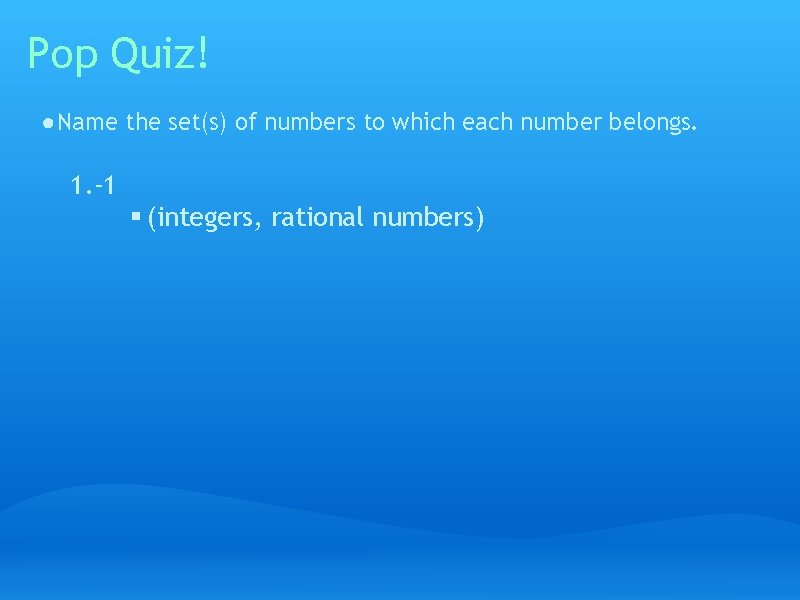Pop Quiz! ● Name the set(s) of numbers to which each number belongs. 1. -1 § (integers, rational numbers)Pop Quiz! ● Name the set(s) of numbers to which each number belongs. 1. -1 § (integers, rational numbers) 2. -4. 8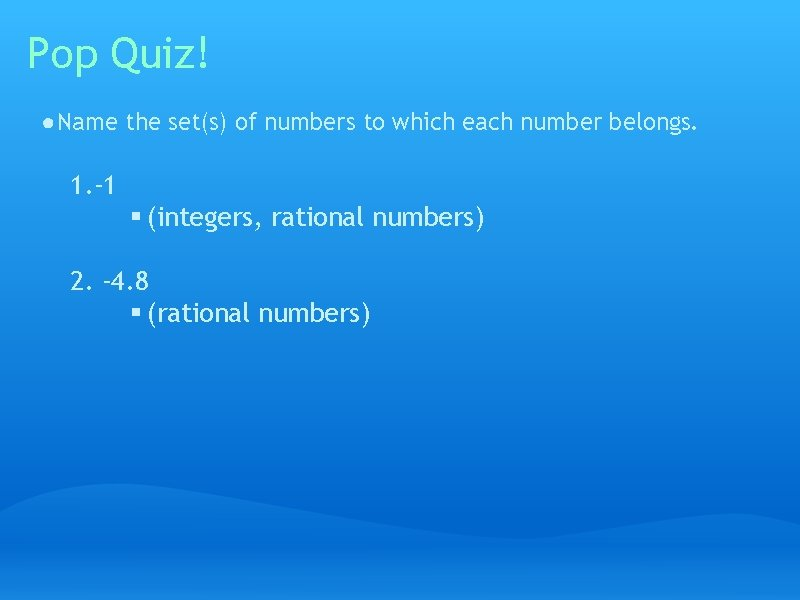Pop Quiz! ● Name the set(s) of numbers to which each number belongs. 1. -1 § (integers, rational numbers) 2. -4. 8 § (rational numbers)Pop Quiz! ● Name the set(s) of numbers to which each number belongs. 1. -1 § (integers, rational numbers) 2. -4. 8 § (rational numbers) 3. -20/4Pop Quiz! ● Name the set(s) of numbers to which each number belongs. 1. -1 § (integers, rational numbers) 2. -4. 8 § (rational numbers) 3. -20/4 § (integers, rational numbers)Pop Quiz! ● Name the set(s) of numbers to which each number belongs. 1. -1 § (integers, rational numbers) 2. -4. 8 § (rational numbers) 3. -20/4 § (integers, rational numbers) 4. 7Pop Quiz! ● Name the set(s) of numbers to which each number belongs. 1. -1 § (integers, rational numbers) 2. -4. 8 § (rational numbers) 3. -20/4 § (integers, rational numbers) 4. 7 § (natural numbers, whole numbers, integers, rational numbers)Exploring Real Numbers THE END